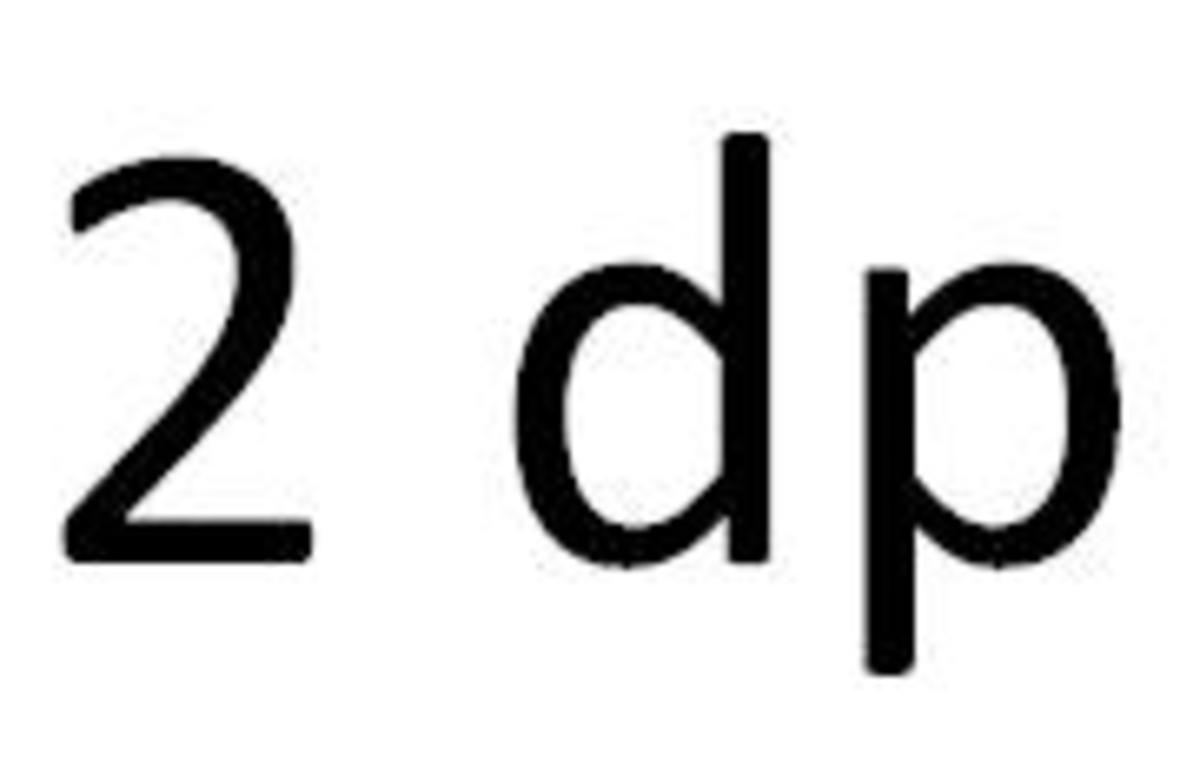Updated date:

# How to round off a number to 2 decimal places (2dp math rounding help)

Author:

## How To Round To 2 Decimal Places VideoIn math exams you may be asked to round your answers off to 2 decimal places, so this hub will show you how to do this. If you are dealing with money problems then the answer will have to be given to the nearest whole or to 2 decimal places.

Example 1

Round 3.863 off to 2 decimal places.

2 decimal places means that the answer needs to have 2 numbers after the decimal point.

Let’s highlight all the numbers up to 2 numbers after the decimal point:

3.862

Now all you need to do next is look at the number after the 6 to decide if you round the number down (keep it the same as 3.86) or up (round to 3.87).

Since the number after the 6 is 2 you round the number down (since 2 is less than 5 – halfway). Therefore the answer is 3.86

Example 2

Round 14.816 off to 2 decimal places.

2 decimal places means that the answer needs to have 2 numbers after the decimal point.

Let’s highlight all the numbers up to 2 numbers after the decimal point:

14.816

Now all you need to do next is look at the number after the 1 to decide if you round the number down (keep it the same as 14.81) or up (round to 14.82).

Since the number after the 1 is 6 you round the number up (since 6 is more than or equal to 5). Therefore the answer is 14.82

Example 3

Round 6.1486713 off to 2 decimal places.

2 decimal places means that the answer needs to have 2 numbers after the decimal point.

Let’s highlight all the numbers up to 2 numbers after the decimal point:

6.1486713

Now all you need to do next is look at the number after the 4 to decide if you round the number down (keep it the same as 6.14) or up (round to 6.15).

Since the number after the 4 is 8 you round the number up (since 8 is more than or equal to 5). Therefore the answer is 6.15

Example 4

Round 174.8029 off to 2 decimal places.

2 decimal places means that the answer needs to have 2 numbers after the decimal point.

Let’s highlight all the numbers up to 2 numbers after the decimal point:

174.8019

Now all you need to do next is look at the number after the 0 to decide if you round the number down (keep it the same as 174.80) or up (round to 174.81).

Since the number after the 0 is 1 you round the number down (since 1 is less than 5). Therefore the answer is 174.80

Example 5

Share \$247 equally between 9 people.

Since the question is money related you need to round the answer off to decimal places:

247 ÷ 9 = 27.444...

Let’s highlight all the numbers up to 2 numbers after the decimal point:

27.444...

Now all you need to do next is look at the number after the 4 to decide if you round the number down (keep it the same as 27.44) or up (round to 27.45).

Since the number after the 4 is 4 you round the number down (since 4 is less than 5). Therefore the answer is \$27.44 to decimal places.

## Comments

bob on October 04, 2011:

what is 8138 rounded to 2 decimal place????